﻿ 基于QR码构造的QC-LDPC码译码器设计与实现文章快速检索 高级检索
 重庆邮电大学学报(自然科学版)2020, Vol. 32Issue (3): 419-425  DOI: 10.3979/j.issn.1673-825X.2020.03.0110

### 引用本文LIU Zhen, LI Yong. Design and implementation of QC-LDPC decoder based on QR code[J]. Journal of Chongqing University of Posts and Telecommunications (Natural Science Edition), 2020, 32(3): 419-425.   DOI: 10.3979/j.issn.1673-825X.2020.03.011.### Foundation item

The National Natural Science Foundation of China (61771081)

.

### 文章历史

1. 重庆邮电大学 通信与信息工程学院，重庆 400065;
2. 重庆大学 计算机学院，重庆 400044

Design and implementation of QC-LDPC decoder based on QR code
LIU Zhen1, LI Yong1,21. School of Communication and Information Engineering, Chongqing University of Posts and Telecommunications, Chongqing 400065, P.R. China;
2. School of Computer Science, Chongqing University, Chongqing 400044, P.R. China
Abstract: The row weight of quasi cyclic low-density parity check (QC-LDPC) codes based on quadratic residue (QR) codes is usually large, which causes that the decoder will consume more resources on hardware implementation. Therefore, this paper designed a layered decoder based on partial-parallel structure compromised between resource occupancy and throughput. The decoder is implemented by the Layered Normalized Min-Sun Algorithm (LNMSA), which simultaneously processes consecutive n rows of a layer using a partial-parallel structure. On the storage structure of variable node posterior probability information, the continuous n information is combined into one group and the consecutive two groups are alternately stored by two random access memories(RAM). When obtaining the minimum value and the second small value, the input message is divided into 4 groups. Then, 4 minimum value are respectively computed from 4 groups and the global minimum value and second small value are obtained from them, which can effectively reduce the complexity of the comparison operation. In the test environment with code length of 2 040, code rate of 0.83 and Xilinx Virtex-6 development board, the throughput of the decoder can reach 447.5 Mbit/s when working on 166.7 MHz.
Keywords: quasi cyclic low-density parity check code    layered min-sum decoding algorithm    decoder
0 引言

1 QC-LDPC码译码算法

LDPC码一般采用置信传播迭代(belief propagation, BP)算法进行译码，但是BP算法有大量的乘法和指数运算。为了降低复杂度，有学者提出了对数域BP算法。然而，对数域BP算法存在一个非线性函数，FPGA实现时会导致性能极大地下降。因此，后来又有学者提出了适合硬件实现的最小和算法(min-sum algorithm, MSA)及其改进算法。

1) 初始化

 $L\left( {{Q_i}} \right) = L\left( {{C_i}} \right)$ (1)

(1) 式中：L(Qi)表示第i个变量节点的初始后验概率信息；L(Ci)表示第i个信道软信息。

2) 变量节点更新

 ${L^{(n,m)}}\left( {{q_{ij}}} \right) = {L^{(n,m - 1)}}\left( {{Q_i}} \right) - {L^{(n - 1,m)}}\left( {{r_{ji}}} \right)$ (2)

(2) 式中，(n, m)表示第n次迭代第m层。

3) 校验节点更新

 ${L^{(n,m)}}\left( {{r_{ji}}} \right) = \alpha \cdot {\lambda _{i'j}} \cdot {\varphi _{i'j}}$ (3)
 ${\lambda _{i'j}} = \prod\limits_{{i^\prime } \in {R_j}\backslash i} {{\mathop{\rm sign}\nolimits} } \left( {L\left( {{q_{{i^\prime }j}}} \right)} \right)$ (4)
 ${\varphi _{i'j}} = \prod\limits_{{i^\prime } \in {R_j}\backslash i} {\min } \left( {L\left( {{q_{{i^\prime }j}}} \right)} \right)$ (5)

(3)—(5)式中，α为修正因子，取值为(0, 1)。

4) 变量节点后验概率更新

 ${L^{(n,m)}}\left( {{Q_i}} \right) = {L^{(n,m)}}\left( {{q_{ij}}} \right) + {L^{(n,m)}}\left( {{r_{ji}}} \right)$ (6)

(6) 式中：L(n, m)(Qi)表示第n次迭代第m层的后验概率信息；L(n, m)(qij)表示第n次迭代第m层的第i个变量节点传递给第j个校验节点的信息；L(n, m)(rji)表示第n次迭代第m层的第j个校验节点传递给第i个变量节点的信息。

5) 译码判决

 ${\hat c_i} = \left\{ {\begin{array}{*{20}{l}} {1,}&{L\left( {{Q_i}} \right) < 0}\\ {0,}&{L\left( {{Q_i}} \right) \ge 0} \end{array}} \right.$ (7)

(7) 式中，${\hat c_i}$表示第i个译码信息。如果$\left( {{{\hat c}_0}\quad {{\hat c}_1}\quad \cdots \;\;\;\;{{\hat c}_i}\;\quad \cdots } \right)$与校验矩阵的转置矩阵的乘积为零向量或者迭代次数达到最大迭代次数则停止迭代，反之返回步骤2)进行迭代。

2 译码器设计

2.1 译码器整体结构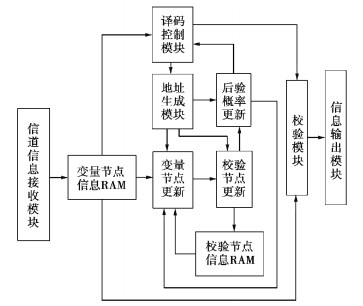图 1 译码器结构框图 Fig.1 Structure diagram of decoder
2.2 信息接收模块及存储结构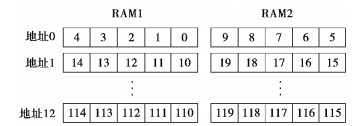图 2 变量节点存储结构 Fig.2 Storage structure of variable node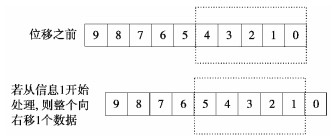图 3 变量节点信息的选取 Fig.3 Chosen of variable node information

2.3 算法模块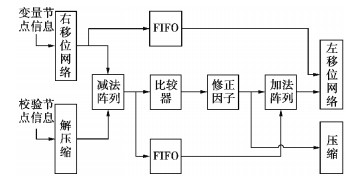图 4 算法模块流水线结构 Fig.4 Pipeline structure of algorithm module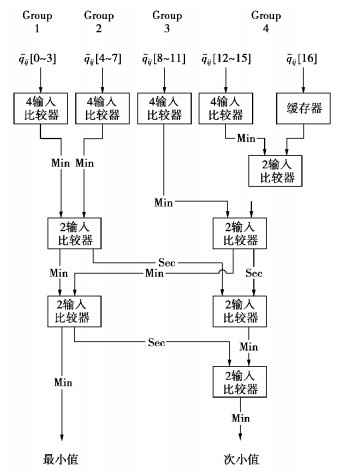图 5 比较器结构 Fig.5 Comparator structure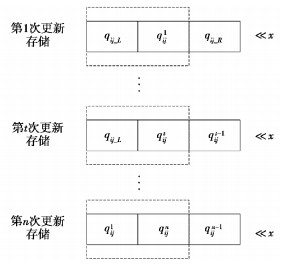图 6 变量节点后验概率信息存储规则 Fig.6 Storage rule of variable node posterior probability

2.4 校验模块

2.5 校验矩阵重排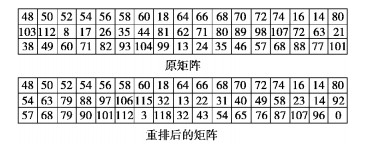图 7 校验矩阵重排 Fig.7 Reordering of parity check matrix
3 译码器的硬件实现表 1 译码器资源消耗 Tab.1 Resource consumption of decoder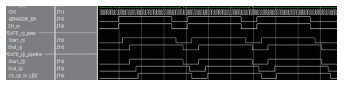图 8 译码器时序图 Fig.8 Decoder timing diagram

 $T = \frac{{f \cdot L}}{{N \cdot I}}$ (8)

(8) 式中：T表示吞吐量；f表示时钟频率；L表示码长；N表示单次迭代所需的时钟周期数；I表示迭代次数。因此，在8次迭代下，由(8)式可知译码器的吞吐量可达447.5 Mbit/s。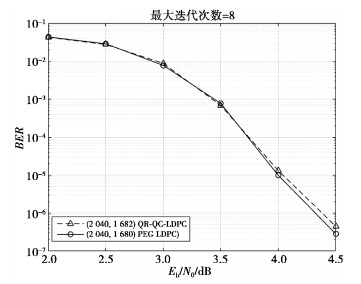图 9 基于QR码构造的QC-LDPC码与PEG算法构造的码字性能对比 Fig.9 Performance comparison of QC-LDPC code based on QR code and LDPC code constructed by PEGalgorithm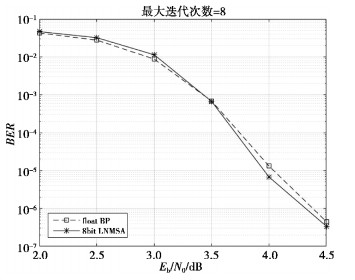图 10 译码器性能对比 Fig.10 Performance comparison of decoder表 2 译码器性能对比 Tab.2 Decoder performance comparison
4 结论

  GALLAGERR. Low-density parity-check codes[J]. IRE Transactions on Information Theory, 1962, 1(8): 21-28.  KOU Y, LIN S, FOSSORIER M P C. Low-density parity-check codes based on finite geometries: a rediscovery and new results[J]. IEEE Trans.on Inform.theory, 2001, 47(7): 2711-2736. DOI:10.1109/18.959255  陈高明, 黎勇, 董灿, 等. 一种(71, 36, 11)QR码的快速代数译码算法[J]. 重庆邮电大学学报(自然科学版), 2015, 27(6): 781-785. CHEN G M, LI Y, DONG C, et al. A fast algebraic decoding algorithm of the (71, 36, 11) quadratic residue code[J]. Journal of Chongqing University of Posts and Telecommunications (Natural Science Edition), 2015, 27(6): 781-785.  李西亚, 黎勇. 基于(17, 9)平方剩余码的广义LDPC码构造及性能研究[J]. 重庆邮电大学学报(自然科学版), 2018, 30(06): 797-802. LI X Y, LI Y. Research on design and performance of GLDPC codes base on (17, 9) quadratic residue codes[J]. Journal of Chongqing University of Posts and Telecommunications (Natural Science Edition), 2018, 30(06): 797-802.  江涛.QC-LDPC码设计和分层译码器的FPGA实现[D].南京: 南京航空航天大学, 2012. JIANG T. Design and implementation of QC-LDPC layered decoder on FPGA[D]. Nanjing: Nanjing University of Aeronautics and Astronautics, 2012.  周健, 吕毅博, 洪少华, 等. 面向磁记录信道的原模图LDPC码译码器的FPGA设计[J]. 重庆邮电大学学报(自然科学版), 2013, 25(6): 788-794. ZHOU J, LV Y B, HONG S H, et al. Protograph-based LDPC decoder applied to magnetic recording channel[J]. Journal of Chongqing University of Posts and Telecommunications (Natural Science Edition), 2013, 25(6): 788-794.  XIANG J. Design and implementation of the high speed LDPC code for 802[J]. 11ad: University of Electronic Science and Technology, 2015.  HAILES P, XU L, MAUNDER R G, et al. A flexible FPGA-based Quasi-Cyclic LDPC decoder[J]. IEEE Access, 2017(5): 20965-20984.  NIDAGUNDI J C and PATIL S R. High throughput structured LDPC layered decoder[C]//2017 International Conference on Wireless Communications, Signal Processing and Networking (WiSPNET). Chennai: IEEE, 2017: 1559-1563.  TSATSARAGKOS I, PALIOURAS V. A reconfigurable LDPC decoder optimized for 802.11n/ac applications[J]. IEEE Transactions on Very Large Scale Integration (VLSI) Systems, 2018, 26(1): 182-195. DOI:10.1109/TVLSI.2017.2752086  袁建国, 汪哲, 何昌伟, 等. 光通信系统中一种改进的LLR-BP译码算法[J]. 半导体光电, 2016, 37(4): 532-535. YUAN J G, WANG Z, HE C W, et al. An improved LLR-BP decoding algorithm for optical communication systems[J]. Semiconductor Optoelectronics, 2016, 37(4): 532-535.  吴淼, 邱丽鹏, 周林, 等. 高效低复杂度的QC-LDPC码全并行分层结构译码器[J]. 重庆邮电大学学报(自然科学版), 2018, 30(3): 354-361. WU M, QIU L P, ZHOU L, et al. Efficient low-complexity full-parallel-layered decoder for QC-LDPC codes[J]. Journal of Chongqing University of Posts and Telecommunications (Natural Science Edition), 2018, 30(3): 354-361.  ZHANG W C, CHEN S, BAI X F, et al. A full layer parallel QC-LDPC decoder for WiMAX and Wi-Fi[C].//2015 IEEE 11th International Conference on ASIC (ASICON). Chengdu: IEEE, 2015: 1-4.  LEE H C, CHENG C C, UENG Y G. Hardware-friendly Probabilistic Min-Sum algorithm for fully-parallel LDPC decoders[C]//2014 8th International Symposium on Turbo Codes and Iterative Information Processing (ISTC). Bremen: IEEE, 2014: 102-106.  KHAZRAIE S, ASVADI R, BANIHASHEMI A H. A PEG Construction of Finite-Length LDPC Codes with Low Error Floor[J]. IEEE Communications Letters, 2012, 16(8): 1288-1291. DOI:10.1109/LCOMM.2012.060112.120844  HUNG S Y, YEN S W, CHEN C H, et al. A 5.7Gbps row-based layered scheduling LDPC decoder for IEEE 802.15.3c applications[C]//2010 IEEE Asian Solid-State Circuits Conference. Beijing: IEEE, 2010: 1-4.  唐兴国.多码率LDPC码编译码器的FPGA实现[D].大连: 大连理工大学, 2013. TANG X G. Implementation of encoder and decoder for multi-rate LDPC codes based on FPGA[D]. Dalian: Dalian University of Technology, 2013. http://cdmd.cnki.com.cn/Article/CDMD-10141-1013199478.htm  彭阳阳, 仰枫帆. 基于FPGA的QC-LDPC码分层译码器设计[J]. 无线电工程, 2014, 44(2): 17-20. PENG Y Y, YANG F F. Design on QC-LDPC layered decoder based on FPGA[J]. Radio Communications Technology, 2014, 44(2): 17-20. DOI:10.3969/j.issn.1003-3106.2014.02.005